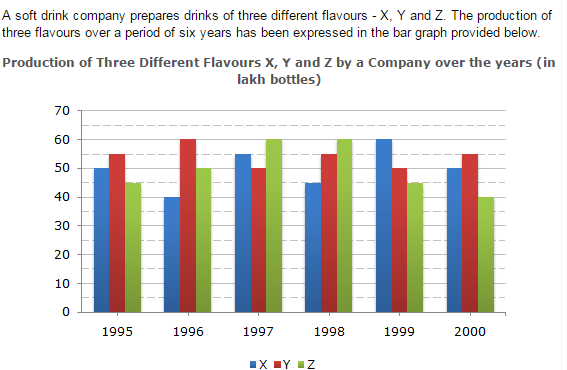Direction1.

The total production of flovour Z in 1997 and 1998 is what percentage of the total production of flavour X in 1995 and 1996?

 A. 96.67% B. 102.25% C. 115.57% D. 133.33%
Explanation: Required percentage = [ (60 + 60) x 100 ] % (50 + 40) = [ 120 x 100 ] % 90 = 133.33%.

2.

For which flavour was the average annual production maximum in the given period?

 A. X only B. Y only C. Z only D. X and Y
Explanation: Average annual productions over the given period for various flavours are: For Flavour X = [ 1 x (50 + 40 + 55 + 45 + 60 + 50) ] = 50 lakh bottles. 6 For Flavour Y = [ 1 x (55 + 60 + 50 + 55 + 50 + 55) ] = 54.17 lakh bottles. 6 For Flavour Z = [ 1 x (45 + 50 + 60 + 60 + 45 + 40) ] = 50 lakh bottles. 6 Therefore Maximum average production is for Flavour Y.

3.

What is the difference between the average production of flavour X in 1995, 1996 and 1997 and the average production of flavour Y in 1998, 1999 and 2000?

 A. 50,000 bottles B. 80,000 bottles C. 2,40,000 bottles D. 5,00,000 bottles
Explanation: Average production of flavour X in 1995, 1996 and 1997 = [ 1 x (50 + 40 + 55) ] 3 = ( 145 ) lakh bottles. 3 Average production of flavour Y in 1998, 1999 and 2000 = [ 1 x (55 + 50 + 55) ] 3 = ( 160 ) lakh bottles. 3 Therefore Difference = ( 160 - 145 ) 3 3 = 15 3 = 5 lakh bottles = 5,00,000 bottles.

4.

What was the approximate decline in the production of flavour Z in 2000 as compared to the production in 1998?

 A. 50% B. 42% C. 33% D. 25%